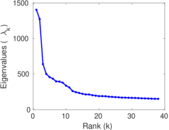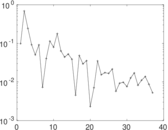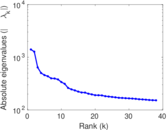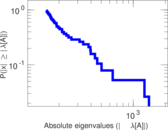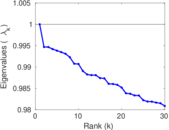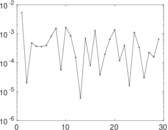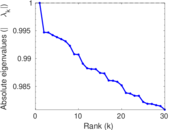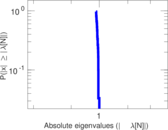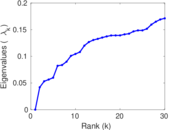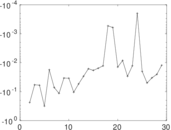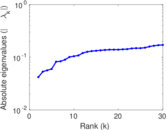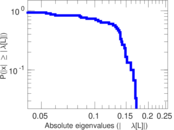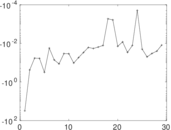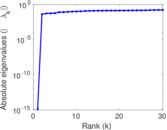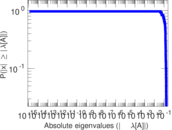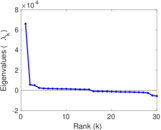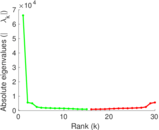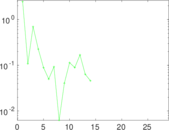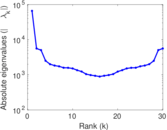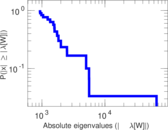# Wikipedia edits (si)

This is the bipartite edit network of the Sinhala Wikipedia. It contains users and pages from the Sinhala Wikipedia, connected by edit events. Each edge represents an edit. The dataset includes the timestamp of each edit.

 Code `si` Internal name `edit-siwiki` Name Wikipedia edits (si) Data source http://dumps.wikimedia.org/ AvailabilityDataset is available for download Consistency checkDataset passed all tests Category Authorship network Dataset timestamp 2017-10-20 Node meaning User, article Edge meaning Edit Network formatBipartite, undirected Edge typeUnweighted, multiple edges Temporal dataEdges are annotated with timestamps

## Statistics

 Size n = 66,095 Left size n1 = 3,945 Right size n2 = 62,150 Volume m = 343,632 Unique edge count m̿ = 140,689 Wedge count s = 341,159,414 Claw count z = 1,173,658,924,192 Square count q = 62,994,873 4-Tour count T4 = 1,868,909,542 Maximum degree dmax = 46,782 Maximum left degree d1max = 46,782 Maximum right degree d2max = 921 Average degree d = 10.398 1 Average left degree d1 = 87.105 7 Average right degree d2 = 5.529 07 Fill p = 0.000 573 815 Average edge multiplicity m̃ = 2.442 49 Size of LCC N = 63,414 Diameter δ = 11 50-Percentile effective diameter δ0.5 = 3.420 70 90-Percentile effective diameter δ0.9 = 3.954 77 Median distance δM = 4 Mean distance δm = 3.767 40 Gini coefficient G = 0.830 322 Balanced inequality ratio P = 0.156 970 Left balanced inequality ratio P1 = 0.088 984 7 Right balanced inequality ratio P2 = 0.221 737 Relative edge distribution entropy Her = 0.741 494 Power law exponent γ = 3.045 41 Tail power law exponent γt = 2.381 00 Tail power law exponent with p γ3 = 2.381 00 p-value p = 0.000 00 Left tail power law exponent with p γ3,1 = 1.791 00 Left p-value p1 = 0.000 00 Right tail power law exponent with p γ3,2 = 2.461 00 Right p-value p2 = 0.000 00 Degree assortativity ρ = −0.291 275 Degree assortativity p-value pρ = 0.000 00 Spectral norm α = 1,404.23 Algebraic connectivity a = 0.041 882 9 Spectral separation |λ1[A] / λ2[A]| = 1.103 34 Controllability C = 57,660 Relative controllability Cr = 0.887 664

## Plots

### Fruchterman–Reingold graph drawing### Degree distribution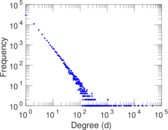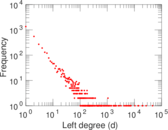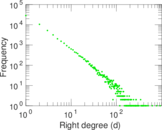### Cumulative degree distribution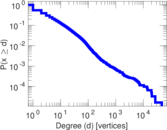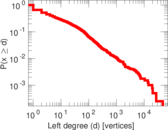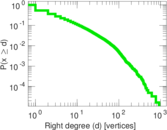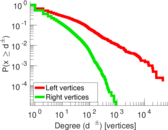### Lorenz curve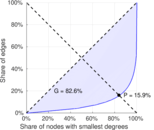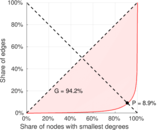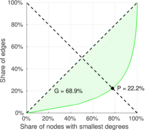### Spectral distribution of the adjacency matrix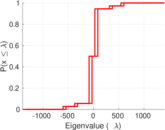### Spectral distribution of the normalized adjacency matrix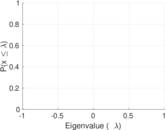### Spectral distribution of the Laplacian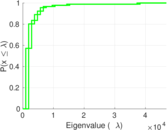### Spectral graph drawing based on the adjacency matrix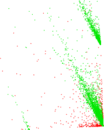### Spectral graph drawing based on the Laplacian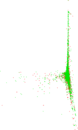### Spectral graph drawing based on the normalized adjacency matrix### Degree assortativity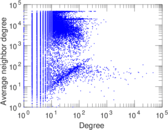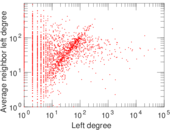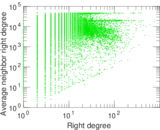### Zipf plot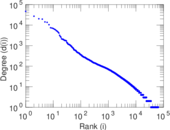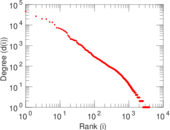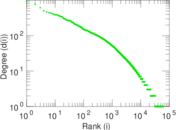### Hop distribution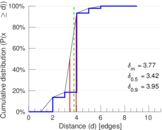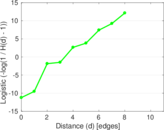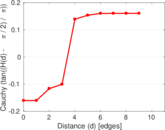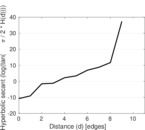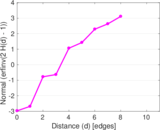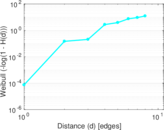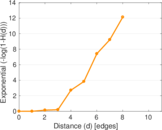### Double Laplacian graph drawing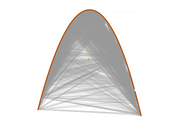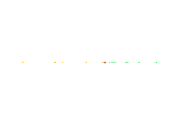### Delaunay graph drawing### Edge weight/multiplicity distribution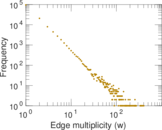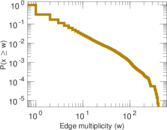### Temporal distribution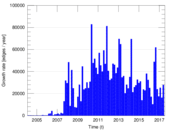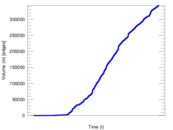### Temporal hop distribution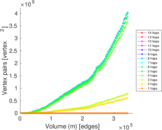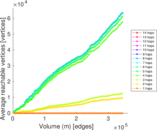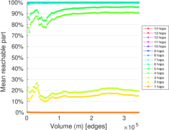### Diameter/density evolution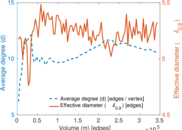### Matrix decompositions plots